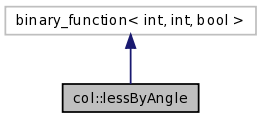# col::lessByAngle Struct Reference

Compare points by angle. More...

Inheritance diagram for col::lessByAngle:[legend]
Collaboration diagram for col::lessByAngle:[legend]
List of all members.

## Public Member Functions

lessByAngle (int xValue, int yValue, vector< Pnt3f > &pointVector)
bool operator() (int i1, int i2) const

## Public Attributes

int x
points will be projected onto that plane
int y
vector< Pnt3f > & point
the points themselves; the () expects to get 2 indices into this array

## Detailed Description

Compare points by angle.

Returns:
True if point[i1] < point[i2] compared by angle, flse otherwise.
Two points are connected with a "center" by a line each, then the angle between those two lines and the `x` axis are computed, and those are compared. This functor is meant as a Binary Predicate for `sort()`.

Angles are in [0,360). No trigonometric function are evaluated, and if the two points are in different quadrants, then only comparisons are made, i.e. / quadrant(p1) < quadrant(p2), p1,p2 in different qu's p1 < p2 <=> | \ p1_y/p1_x < p2_y/p2_x , p1,p2 in same quadrant

Precondition:
`x`, `y`, and `point`,npoints are set.
Implementation:
The functor could return -1/0/+1 for less-than/euqal/greater-than. The original of this function is opyCompPointsByAngle in Y.

The documentation for this struct was generated from the following file:
Generated on Tue Oct 16 18:13:10 2007 for CollDet by1.5.2